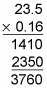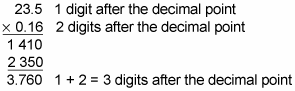Multiplying decimals is different from adding and subtracting them in that you don’t have to worry about lining up the decimal points. In fact, the only difference between multiplying whole numbers and decimals comes at the very end.

Here’s how to multiply decimals:

1. Perform the multiplication as you would for whole numbers.

2. When you’re done, count the number of digits to the right of the decimal point in each factor and add the result.

This process sounds tricky, but multiplying decimals can actually be simpler than adding or subtracting them. Suppose, for instance, you want to multiply 23.5 by 0.16. The first step is to pretend that you’re multiplying numbers without decimal points:This answer isn’t complete, though, because you still need to find out where the decimal point goes. To do this, notice that 23.5 has one digit after the decimal point and that 0.16 has two digits after the decimal point. Because 1 + 2 = 3, place the decimal point in the answer so that it has three digits after the decimal point. (You can put your pencil at the 0 at the end of 3760 and move the decimal point three places to the left.)Even though the last digit in the answer is a 0, you still need to count this as a digit when placing the decimal point. After the decimal point is in place, you can drop trailing zeros.

So the answer is 3.760, which is equal to 3.76.